# Monodromy group

Jump to: navigation, search

## Definition

Suppose$X$ is a path-connected locally path-connected topological space and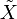$\tilde{X}$ is a covering space of$X$ with covering map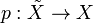$p:\tilde{X} \to X$. Suppose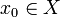$x_0\in X$. Consider the set$p^{-1}(x_0)$. There is a natural group action of the fundamental group$\pi_1(X,x_0)$ on the set$p^{-1}(x_0)$ defined as follows:

Any loop starting and ending at$x_0$ defines, for each point in$p^{-1}(x_0)$, a unique path starting at the point. Consider the endpoint of this path. This gives a set map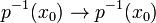$p^{-1}(x_0) \to p^{-1}(x_0)$. The inverse loop gives the inverse set map, so the set map is a permutation. Thus, for each loop, we get an element of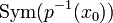$\operatorname{Sym}(p^{-1}(x_0))$. In other words, we have a map: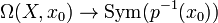$\Omega(X,x_0) \to \operatorname{Sym}(p^{-1}(x_0))$

Two homotopic loops (in the based homotopy sense) induce the same permutation, so this descends to a map: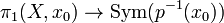$\pi_1(X,x_0) \to \operatorname{Sym}(p^{-1}(x_0))$

Finally, we can verify that the map is a group homomorphism.

The image of this map, viewed as a subgroup of$\operatorname{Sym}(p^{-1}(x_0))$ (i.e., not just as an abstract group, but in the context of the action) is termed the monodromy group for the covering map at$x_0$. The group action itself is termed the monodromy action.